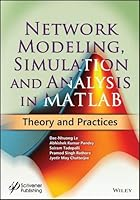# Network Modeling, Simulation and Analysis in MATLAB: Theory and Practices## Book Description

The purpose of this book is first to study MATLAB concepts, then the basic concepts of and simulation , particularly focus on digital simulation. The book will cover the topics practically to describe routing simulation using MATLAB tool. It will cover the dimensions’ like and WSN simulation using MATLAB, then depict the modeling and simulation of vehicles power in detail along with considering different case studies.

Key features of the book include:

• Discusses different basics and advanced methodology with their fundamental concepts of exploration and exploitation in NETWORK SIMULATION.
• Elaborates practice questions and simulations in MATLAB
• Student-friendly and Concise
• Useful for UG and PG level research scholar
• Aimed at Practical approach for network simulation with more programs with step by step comments.
• Based on the Latest technologies, coverage of wireless simulation and WSN concepts and implementations